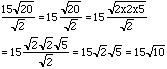Date: Sat, 12 Sep 1998 09:59:58 -0600 (CST)
Subject: Approximation Of Radicals

Name: Lana Sabo

Who is asking: Student
Level: Secondary

Question:
fifteen times the square root of twenty, divided by the square root of 2.

nine subtract the square root of forty-five, divided by 3.

the square root of 18 plus the square root of 12, divided by the square root of 3.

Hi Lana
Expressions that involve radicals can sometimes be simplified. For example your first question
fifteen times the square root of twenty, divided by the square root of 2I hope this helps
Harley

Go to Math Central

To return to the previous page use your browser's back button.